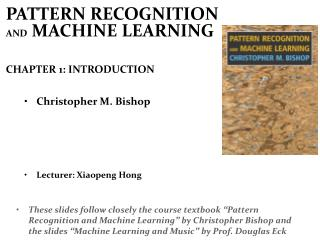# Christopher M. Bishop - PowerPoint PPT PresentationDownload PresentationChristopher M. Bishop

Christopher M. BishopDownload Presentation## Christopher M. Bishop

- - - - - - - - - - - - - - - - - - - - - - - - - - - E N D - - - - - - - - - - - - - - - - - - - - - - - - - - -
##### Presentation Transcript

1. PATTERN RECOGNITION AND MACHINE LEARNING CHAPTER 1: INTRODUCTION • Christopher M. Bishop • Lecturer: Xiaopeng Hong • These slides follow closely the course textbook “Pattern Recognition and Machine Learning” by Christopher Bishop and the slides “Machine Learning and Music” by Prof. Douglas Eck

2. 免责声明

3. ML IP SP Contents CV PR Pattern Classification Feature Extraction Statistical Symbol Probability Theory Information Theory Mathematical logic …

4. PR & ML • Pattern recognition has its origins in engineering, whereas machine learning grew out of computer science. However, these activities can be viewed as two facets of the same field, and together they have undergone substantial development over the past ten years.

5. Learning • Learning denotes changes in the system that is adaptive in the sense that they enable the system to do the same task or tasks drawn from the same population more effectively the next time. • by H. Simon • 如果一个系统能够通过执行某种过程而改进它的性能，这就是学习。 by 陆汝钤教授

6. Conference ICML KDD NIPS IJCNN AIML IJCAI COLT CVPR ICCV ECCV … Journal Machine Learning (ML) Journal of Machine Learning Research Annals of Statistics Data Mining and Knowledge Discovery IEEE-KDE IEEE-PAMI Artificial Intelligence Journal of Artificial Intelligence Research Computational Intelligence Neural Computation IEEE-NN Research, Information and Computation … Related Publications

7. Classical statistical methods Division in feature space PAC Generalization Ensemble learning History BP Network F. Rosenblatt. Perceptron M. Minsky. “Perceptron”

8. Leslie Gabriel Valiant • He introduced the "probably approximately correct" (PAC) model of machine learning that has helped the field of computational learning theory grow. by Wikipedia • 将计算复杂性作为一个必须考虑的因素。算法的复杂性必须是多项式的。为了达到这个目的，不惜牺牲模型精度。 • “对任意正数ε>0，0≤δ<1，|F(x)-f(x)|≤ε成立的概率大于1-δ” • 对这个理念，传统统计学家难以接受 by 王珏教授

9. Vladimir N. Vapnik • “不能将估计概率密度这个更为困难的问题作为解决机器学习分类或回归问题的中间步骤，因此，他直接将问题变为线性判别问题其本质是放弃机器学习建立的模型对自然模型的可解释性。” • “泛化”“有限样本统计” • 泛化作为机器学习的核心问题 • 在线性特征空间上设计算法 • 泛化最大边缘 • “与其一无所有，不如求其次”。这是统计学的传统无法接受的 by 王珏教授

10. Robert Schapire • “对任意正数ε>0，0≤δ<1，|F(x)-f(x)|≤ε成立的概率大于1/2 + δ” • 构造性证明了 PAC弱可学习的充要条件是PAC强可学习 • 集群学习有两个重要的特点： • 使用多个弱模型代替一个强模型 • 决策方法是以弱模型投票，并以少数服从多数的原则决定解答。 by 王珏教授

11. Example Handwritten Digit Recognition 28 28 d=784 Pre-processing  feature extraction 1. reduce variability ; 2. speed up computation

12. Polynomial Curve Fitting

13. Sum-of-Squares Error Function

14. 0th Order Polynomial

15. 1st Order Polynomial

16. 3rd Order Polynomial

17. 9th Order Polynomial

18. Over-fitting Root-Mean-Square (RMS) Error:

19. Polynomial Coefficients

20. Data Set Size: 9th Order Polynomial

21. Data Set Size: 9th Order Polynomial

22. Regularization Penalize large coefficient values

23. Regularization:

24. Regularization:

25. Regularization: vs.

26. Polynomial Coefficients

27. Probability Theory 统计机器学习/模式分类问题 可以在 贝叶斯的框架下表示 We now seek a more principled approach to solving problems in pattern recognition by turning to a discussion of probability theory. As well as providing the foundation for nearly all of the subsequent developments in this book. ML MAP Bayesian

28. Probability Theory Apples and Oranges

29. Probability Theory Joint Probability Marginal Probability Conditional Probability

30. Probability Theory Product Rule Sum Rule

31. The Rules of Probability Sum Rule Product Rule

32. Bayes’ Theorem posterior  likelihood × prior

33. Probability Densities

34. Transformed Densities

35. Expectations Conditional Expectation (discrete) Approximate Expectation (discrete and continuous)

36. Variances and Covariances

37. The Gaussian Distribution

38. Gaussian Mean and Variance

39. The Multivariate Gaussian

40. Gaussian Parameter Estimation Likelihood function